# Circle + unit conversion - math problems

#### Number of problems found: 78

• Minute handWhat is the distance the minute hand of the clock travels in 12 minutes, if the diameter of the clock is 30 cm and the hand extends to a distance of 2 cm from the edge of the clock?
• A bicycle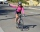A bicycle wheel has a diameter of 63 cm. Calculate how many times the wheel turns round in travelling 19.8 km .
• A bug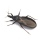A bug was sitting on the tip of a wind turbine blade that was 24 inches long when it started to rotate. The bug held on for five rotations before flying away. How far did the bug travel before it flew off? Exact answer.
• The central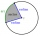The central angle of a sector is 30° and the radius is 15 m. Determine its perimeter.
• Constant Angular Acceleration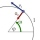The particle began to move from rest along a circle with a constant angular acceleration. After five cycles (n = 5), its angular velocity reached the value ω = 12 rad/s. Calculate the magnitude of the angular acceleration ε of this motion and the time int
• GearsThe front gear on the bike has 32 teeth and the rear, on the wheel, has 12 teeth. How many times does the bike's rear-wheel turn if you turn the right pedal 30 times? What distance will you go if the circumference of the bicycle wheel is 250 cm?
• Central angleWhat is the length of the arc of a circle with a diameter of 46 cm, which belongs to a central angle of 30°?
• Annulus from triangleCalculate the content of the area bounded by a circle circumscribed and a circle inscribed by a triangle with sides a = 25mm, b = 29mm, c = 36mm
• The bridge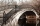A vehicle weighing 5,800 kg passes 41 km/h on an arched bridge with a radius of curvature of 62 m. What force is pushing the car onto the bridge as it passes through the center? What is the maximum speed it can cross over the center of the bridge so that
• Rotaty motionWhat is the minimum speed and frequency that we need to rotate with water can in a vertical plane along a circle with a radius of 70 cm to prevent water from spilling?
• Circular railwayThe railway connects in a circular arc the points A, B, and C, whose distances are | AB | = 30 km, AC = 95 km, BC | = 70 km. How long will the track from A to C?
• Propeller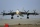The aircraft propeller rotates at an angular speed of 200 rad/s. A) What is the speed at the tip of the propeller if its distance from the axis of rotation is 1.5 m? B) What path does the aircraft travel during one revolution of the propeller at a speed o
• RPMAn electric motor makes 3,000 revolutions per minutes. How many degrees does it rotate in one second?
• Velocipedes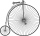In the 19th century, bicycles did not have a chain drive, and the wheel axis connected the pedals directly. This wheel diameter gradually increased until the so-called high bikes (velocipedes) with a front-wheel diameter of up to 1.5 meters, while the rea
• Special watchFero bought a special watch on the market. They have only one (minute) hand and a display that shows which angle between the hour and minute hand. How many hours was his watch shown - the minute hand points to number 2; the display shows 125°?
• DecagonCalculate the area and circumference of the regular decagon when its radius of a circle circumscribing is R = 1m
• The small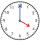The small hand of the wall clock is 15 cm long. What a long journey the end of the hour hand in 15 minutes will describe. (b - in 2 hours)?
• Around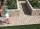Around the circular flowerbed with a radius of 2 m is a sidewalk 80 cm wide. How many square meters does the sidewalk have?
• Bike wheel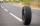The bike wheel has a radius of 30cm. How many times does it turn if we go on a 471m bike?
• ClocksThe length of the minute hand is 12 cm. What track in meters will its end point describe in a week?

Do you have an exciting math question or word problem that you can't solve? Ask a question or post a math problem, and we can try to solve it.

We will send a solution to your e-mail address. Solved examples are also published here. Please enter the e-mail correctly and check whether you don't have a full mailbox.

Circle Problems. Unit conversion - math problems.